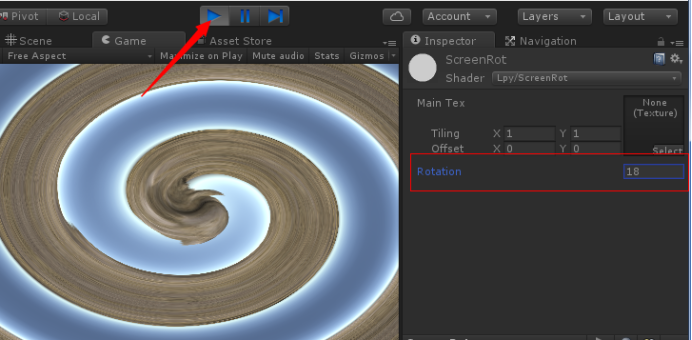x1 = 0.5 + distance *cos(angle)

y1 = 0.5 + distance *sin(angle)

``````Shader "Lpy/ScreenRot"
{
Properties
{
_MainTex ("Main Tex", 2D) = "white" {}
_Rot ("Rotation", float) = 0
}

{
Tags {"Queue"="Geometry"}
Pass
{
Tags { "LightMode"="ForwardBase" }
ZWrite Off

CGPROGRAM
#pragma vertex vert
#pragma fragment frag
#include "UnityCG.cginc"
#define PI 3.14159265358979

sampler2D _MainTex;
float _Rot;
struct a2v
{
float4 vertex : POSITION;
float3 texcoord : TEXCOORD0;
};

struct v2f
{
float4 pos : SV_POSITION;
float2 uv : TEXCOORD0;
};

v2f vert (a2v v)
{
v2f o;
o.pos = mul(UNITY_MATRIX_MVP, v.vertex);
o.uv = v.texcoord;
return o;
}

fixed4 frag (v2f i) : SV_Target
{
//与中心点(0.5,0.5)的距离
float distance = sqrt((i.uv.x - 0.5)*(i.uv.x - 0.5) +(i.uv.y - 0.5)*(i.uv.y - 0.5));
//距离越大，旋转角度越大
_Rot *=distance;
//计算旋转角度
float angle = step(i.uv.x,0.5)*PI+ atan((i.uv.y - 0.5)/(i.uv.x - 0.5)) + _Rot;
//计算坐标
i.uv.x = 0.5 +   distance *cos(angle);
i.uv.y = 0.5 +   distance *sin(angle);

fixed4 c = tex2D(_MainTex, i.uv);
return c;
}
ENDCG
}
}
FallBack "Specular"
}``````

## 2、使用材质

``````using UnityEngine;
using System.Collections;

public class ScreenRot : MonoBehaviour
{
public Material mtl;

void OnRenderImage(RenderTexture src, RenderTexture dest)
{
Graphics.Blit (src, dest,mtl);
}
}``````

## 3、代码中引用

``````using UnityEngine;
using System.Collections;

public class ScreenRot : MonoBehaviour
{
public Material mtl;
public  float rot;

// Update is called once per frame
void Update ()
{
rot += 0.1f;
}

void OnRenderImage(RenderTexture src, RenderTexture dest)
{
if (rot == 0.0)
return;
mtl.SetFloat("_Rot", rot);
Graphics.Blit (src, dest,mtl);
}
}``````

Unity3D热更新框架教程：zhuanlan.zhihu.com/p/21